Open in App
Not now

# Insertion in an AVL Tree

• Difficulty Level : Hard
• Last Updated : 11 Nov, 2022

## AVL Tree:

AVL tree is a self-balancing Binary Search Tree (BST) where the difference between heights of left and right subtrees cannot be more than one for all nodes.

### Example of AVL Tree: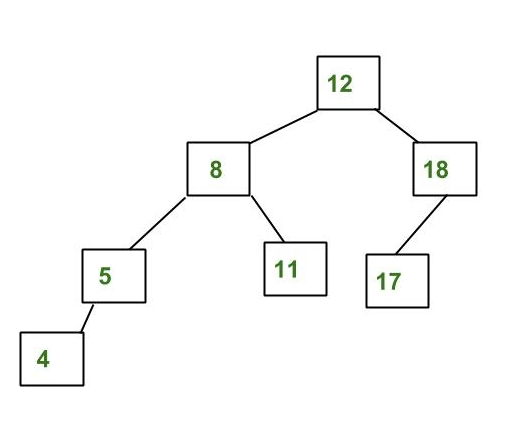The above tree is AVL because the differences between the heights of left and right subtrees for every node are less than or equal to 1.

### Example of a Tree that is NOT an AVL Tree: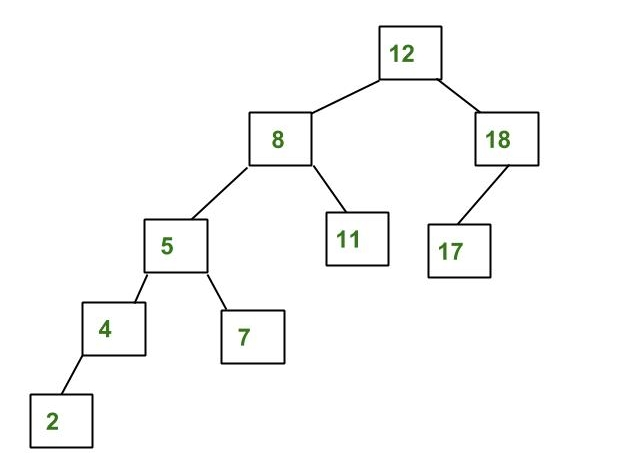The above tree is not AVL because the differences between the heights of the left and right subtrees for 8 and 12 are greater than 1.

## Why AVL Trees?

Most of the BST operations (e.g., search, max, min, insert, delete.. etc) take O(h) time where h is the height of the BST. The cost of these operations may become O(n) for a skewed Binary tree. If we make sure that the height of the tree remains O(log(n)) after every insertion and deletion, then we can guarantee an upper bound of O(log(n)) for all these operations. The height of an AVL tree is always O(log(n)) where n is the number of nodes in the tree.

## Insertion in AVL Tree:

To make sure that the given tree remains AVL after every insertion, we must augment the standard BST insert operation to perform some re-balancing.
Following are two basic operations that can be performed to balance a BST without violating the BST property (keys(left) < key(root) < keys(right)).

• Left Rotation
• Right Rotation
```T1, T2 and T3 are subtrees of the tree, rooted with y (on the left side) or x (on the right side)

y                               x
/ \     Right Rotation          /  \
x   T3   - - - - - - - >        T1   y
/ \       < - - - - - - -            / \
T1  T2     Left Rotation            T2  T3

Keys in both of the above trees follow the following order
keys(T1) < key(x) < keys(T2) < key(y) < keys(T3)
So BST property is not violated anywhere.```
Recommended Practice

### Steps to follow for insertion:

Let the newly inserted node be w

• Perform standard BST insert for w
• Starting from w, travel up and find the first unbalanced node. Let z be the first unbalanced node, y be the child of z that comes on the path from w to z and x be the grandchild of z that comes on the path from w to z
• Re-balance the tree by performing appropriate rotations on the subtree rooted with z. There can be 4 possible cases that need to be handled as x, y and z can be arranged in 4 ways.
• Following are the possible 4 arrangements:
• y is the left child of z and x is the left child of y (Left Left Case)
• y is the left child of z and x is the right child of y (Left Right Case)
• y is the right child of z and x is the right child of y (Right Right Case)
• y is the right child of z and x is the left child of y (Right Left Case)

Following are the operations to be performed in above mentioned 4 cases. In all of the cases, we only need to re-balance the subtree rooted with z and the complete tree becomes balanced as the height of the subtree (After appropriate rotations) rooted with z becomes the same as it was before insertion.

1. Left Left Case

```T1, T2, T3 and T4 are subtrees.
z                                      y
/ \                                   /   \
y   T4      Right Rotate (z)          x      z
/ \          - - - - - - - - ->      /  \    /  \
x   T3                               T1  T2  T3  T4
/ \
T1   T2```

2. Left Right Case

```     z                               z                           x
/ \                            /   \                        /  \
y   T4  Left Rotate (y)        x    T4  Right Rotate(z)    y      z
/ \      - - - - - - - - ->    /  \      - - - - - - - ->  / \    / \
T1   x                          y    T3                    T1  T2 T3  T4
/ \                        / \
T2   T3                    T1   T2```

3. Right Right Case

```  z                                y
/  \                            /   \
T1   y     Left Rotate(z)       z      x
/  \   - - - - - - - ->    / \    / \
T2   x                     T1  T2 T3  T4
/ \
T3  T4```

4. Right Left Case

```   z                            z                            x
/ \                          / \                          /  \
T1   y   Right Rotate (y)    T1   x      Left Rotate(z)   z      y
/ \  - - - - - - - - ->     /  \   - - - - - - - ->  / \    / \
x   T4                      T2   y                  T1  T2  T3  T4
/ \                              /  \
T2   T3                           T3   T4```

### Illustration of Insertion at AVL Tree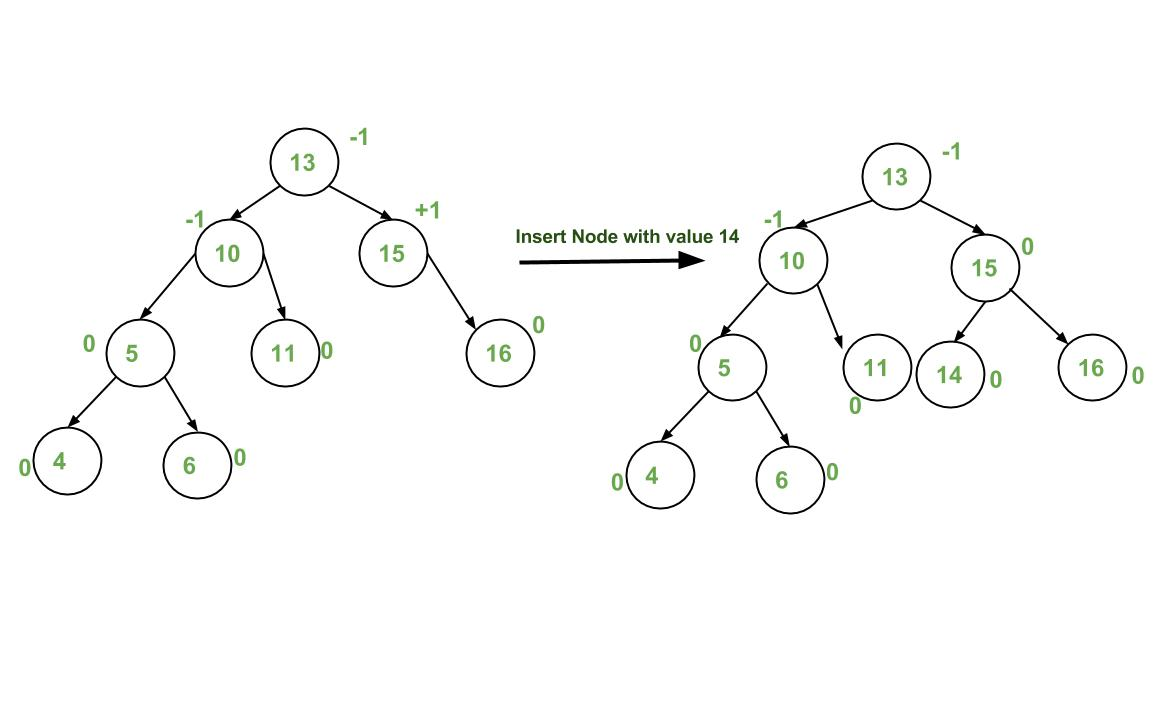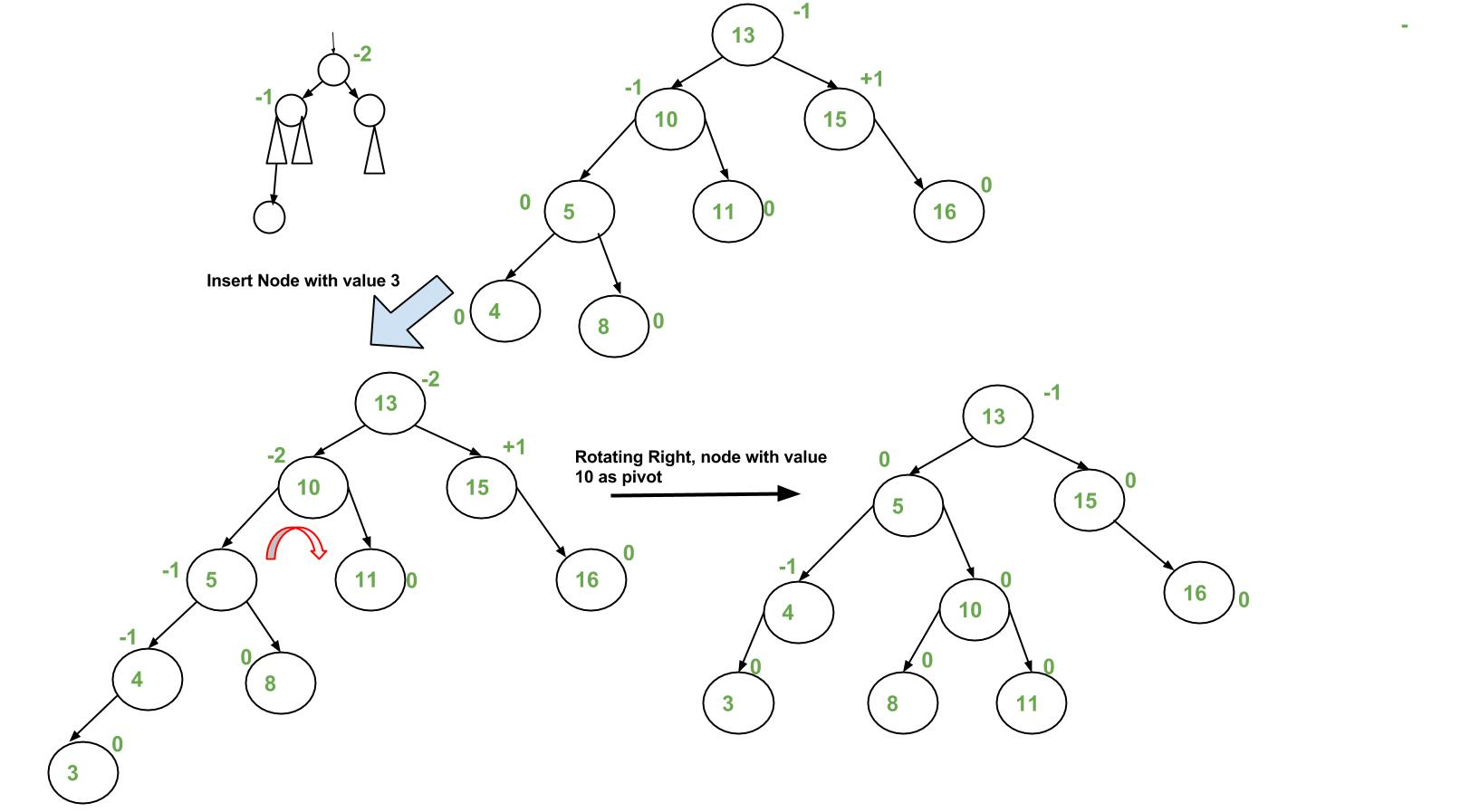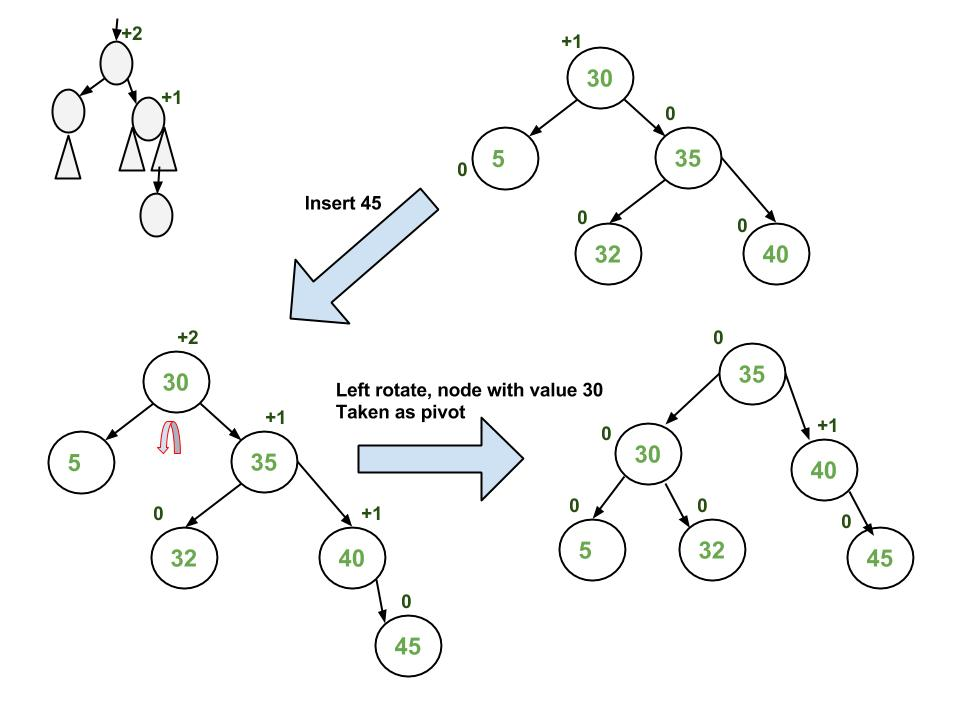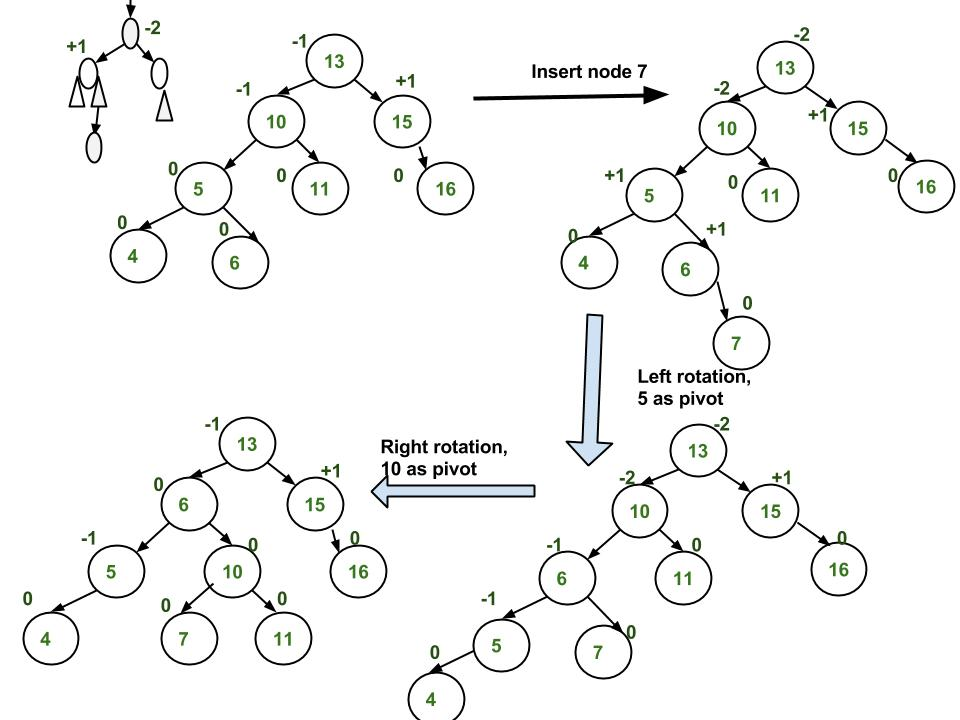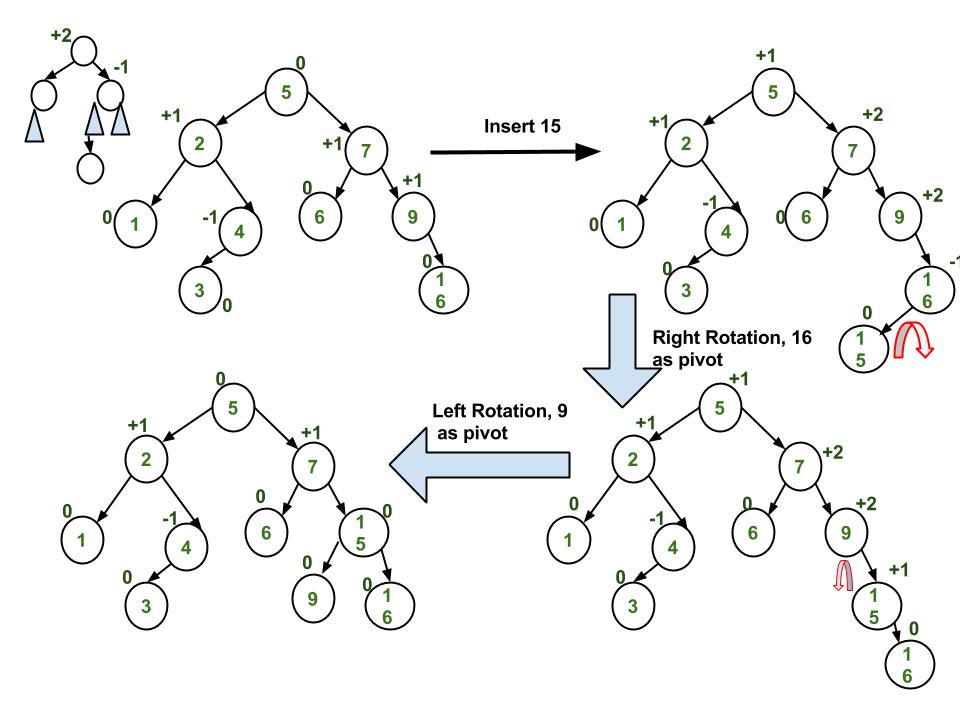### Approach:

The idea is to use recursive BST insert, after insertion, we get pointers to all ancestors one by one in a bottom-up manner. So we don’t need a parent pointer to travel up. The recursive code itself travels up and visits all the ancestors of the newly inserted node.

Follow the steps mentioned below to implement the idea:

• Perform the normal BST insertion.
• The current node must be one of the ancestors of the newly inserted node. Update the height of the current node.
• Get the balance factor (left subtree height – right subtree height) of the current node.
• If the balance factor is greater than 1, then the current node is unbalanced and we are either in the Left Left case or left Right case. To check whether it is left left case or not, compare the newly inserted key with the key in the left subtree root
• If the balance factor is less than -1, then the current node is unbalanced and we are either in the Right Right case or Right-Left case. To check whether it is the Right Right case or not, compare the newly inserted key with the key in the right subtree root.

Below is the implementation of the above approach:

## C++14

 `// C++ program to insert a node in AVL tree``#include``using` `namespace` `std;` `// An AVL tree node``class` `Node``{``    ``public``:``    ``int` `key;``    ``Node *left;``    ``Node *right;``    ``int` `height;``};` `// A utility function to get the``// height of the tree``int` `height(Node *N)``{``    ``if` `(N == NULL)``        ``return` `0;``    ``return` `N->height;``}` `// A utility function to get maximum``// of two integers``int` `max(``int` `a, ``int` `b)``{``    ``return` `(a > b)? a : b;``}` `/* Helper function that allocates a``   ``new node with the given key and``   ``NULL left and right pointers. */``Node* newNode(``int` `key)``{``    ``Node* node = ``new` `Node();``    ``node->key = key;``    ``node->left = NULL;``    ``node->right = NULL;``    ``node->height = 1; ``// new node is initially``                      ``// added at leaf``    ``return``(node);``}` `// A utility function to right``// rotate subtree rooted with y``// See the diagram given above.``Node *rightRotate(Node *y)``{``    ``Node *x = y->left;``    ``Node *T2 = x->right;` `    ``// Perform rotation``    ``x->right = y;``    ``y->left = T2;` `    ``// Update heights``    ``y->height = max(height(y->left),``                    ``height(y->right)) + 1;``    ``x->height = max(height(x->left),``                    ``height(x->right)) + 1;` `    ``// Return new root``    ``return` `x;``}` `// A utility function to left``// rotate subtree rooted with x``// See the diagram given above.``Node *leftRotate(Node *x)``{``    ``Node *y = x->right;``    ``Node *T2 = y->left;` `    ``// Perform rotation``    ``y->left = x;``    ``x->right = T2;` `    ``// Update heights``    ``x->height = max(height(x->left),   ``                    ``height(x->right)) + 1;``    ``y->height = max(height(y->left),``                    ``height(y->right)) + 1;` `    ``// Return new root``    ``return` `y;``}` `// Get Balance factor of node N``int` `getBalance(Node *N)``{``    ``if` `(N == NULL)``        ``return` `0;``    ``return` `height(N->left) - height(N->right);``}` `// Recursive function to insert a key``// in the subtree rooted with node and``// returns the new root of the subtree.``Node* insert(Node* node, ``int` `key)``{``    ``/* 1. Perform the normal BST insertion */``    ``if` `(node == NULL)``        ``return``(newNode(key));` `    ``if` `(key < node->key)``        ``node->left = insert(node->left, key);``    ``else` `if` `(key > node->key)``        ``node->right = insert(node->right, key);``    ``else` `// Equal keys are not allowed in BST``        ``return` `node;` `    ``/* 2. Update height of this ancestor node */``    ``node->height = 1 + max(height(node->left),``                        ``height(node->right));` `    ``/* 3. Get the balance factor of this ancestor``        ``node to check whether this node became``        ``unbalanced */``    ``int` `balance = getBalance(node);` `    ``// If this node becomes unbalanced, then``    ``// there are 4 cases` `    ``// Left Left Case``    ``if` `(balance > 1 && key < node->left->key)``        ``return` `rightRotate(node);` `    ``// Right Right Case``    ``if` `(balance < -1 && key > node->right->key)``        ``return` `leftRotate(node);` `    ``// Left Right Case``    ``if` `(balance > 1 && key > node->left->key)``    ``{``        ``node->left = leftRotate(node->left);``        ``return` `rightRotate(node);``    ``}` `    ``// Right Left Case``    ``if` `(balance < -1 && key < node->right->key)``    ``{``        ``node->right = rightRotate(node->right);``        ``return` `leftRotate(node);``    ``}` `    ``/* return the (unchanged) node pointer */``    ``return` `node;``}` `// A utility function to print preorder``// traversal of the tree.``// The function also prints height``// of every node``void` `preOrder(Node *root)``{``    ``if``(root != NULL)``    ``{``        ``cout << root->key << ``" "``;``        ``preOrder(root->left);``        ``preOrder(root->right);``    ``}``}` `// Driver Code``int` `main()``{``    ``Node *root = NULL;``    ` `    ``/* Constructing tree given in``    ``the above figure */``    ``root = insert(root, 10);``    ``root = insert(root, 20);``    ``root = insert(root, 30);``    ``root = insert(root, 40);``    ``root = insert(root, 50);``    ``root = insert(root, 25);``    ` `    ``/* The constructed AVL Tree would be``                ``30``            ``/ \``            ``20 40``            ``/ \ \``        ``10 25 50``    ``*/``    ``cout << ``"Preorder traversal of the "``            ``"constructed AVL tree is \n"``;``    ``preOrder(root);``    ` `    ``return` `0;``}` `// This code is contributed by``// rathbhupendra`

## C

 `// C program to insert a node in AVL tree``#include``#include` `// An AVL tree node``struct` `Node``{``    ``int` `key;``    ``struct` `Node *left;``    ``struct` `Node *right;``    ``int` `height;``};` `// A utility function to get the height of the tree``int` `height(``struct` `Node *N)``{``    ``if` `(N == NULL)``        ``return` `0;``    ``return` `N->height;``}` `// A utility function to get maximum of two integers``int` `max(``int` `a, ``int` `b)``{``    ``return` `(a > b)? a : b;``}` `/* Helper function that allocates a new node with the given key and``    ``NULL left and right pointers. */``struct` `Node* newNode(``int` `key)``{``    ``struct` `Node* node = (``struct` `Node*)``                        ``malloc``(``sizeof``(``struct` `Node));``    ``node->key   = key;``    ``node->left   = NULL;``    ``node->right  = NULL;``    ``node->height = 1;  ``// new node is initially added at leaf``    ``return``(node);``}` `// A utility function to right rotate subtree rooted with y``// See the diagram given above.``struct` `Node *rightRotate(``struct` `Node *y)``{``    ``struct` `Node *x = y->left;``    ``struct` `Node *T2 = x->right;` `    ``// Perform rotation``    ``x->right = y;``    ``y->left = T2;` `    ``// Update heights``    ``y->height = max(height(y->left),``                    ``height(y->right)) + 1;``    ``x->height = max(height(x->left),``                    ``height(x->right)) + 1;` `    ``// Return new root``    ``return` `x;``}` `// A utility function to left rotate subtree rooted with x``// See the diagram given above.``struct` `Node *leftRotate(``struct` `Node *x)``{``    ``struct` `Node *y = x->right;``    ``struct` `Node *T2 = y->left;` `    ``// Perform rotation``    ``y->left = x;``    ``x->right = T2;` `    ``//  Update heights``    ``x->height = max(height(x->left),  ``                    ``height(x->right)) + 1;``    ``y->height = max(height(y->left),``                    ``height(y->right)) + 1;` `    ``// Return new root``    ``return` `y;``}` `// Get Balance factor of node N``int` `getBalance(``struct` `Node *N)``{``    ``if` `(N == NULL)``        ``return` `0;``    ``return` `height(N->left) - height(N->right);``}` `// Recursive function to insert a key in the subtree rooted``// with node and returns the new root of the subtree.``struct` `Node* insert(``struct` `Node* node, ``int` `key)``{``    ``/* 1.  Perform the normal BST insertion */``    ``if` `(node == NULL)``        ``return``(newNode(key));` `    ``if` `(key < node->key)``        ``node->left  = insert(node->left, key);``    ``else` `if` `(key > node->key)``        ``node->right = insert(node->right, key);``    ``else` `// Equal keys are not allowed in BST``        ``return` `node;` `    ``/* 2. Update height of this ancestor node */``    ``node->height = 1 + max(height(node->left),``                        ``height(node->right));` `    ``/* 3. Get the balance factor of this ancestor``          ``node to check whether this node became``          ``unbalanced */``    ``int` `balance = getBalance(node);` `    ``// If this node becomes unbalanced, then``    ``// there are 4 cases` `    ``// Left Left Case``    ``if` `(balance > 1 && key < node->left->key)``        ``return` `rightRotate(node);` `    ``// Right Right Case``    ``if` `(balance < -1 && key > node->right->key)``        ``return` `leftRotate(node);` `    ``// Left Right Case``    ``if` `(balance > 1 && key > node->left->key)``    ``{``        ``node->left =  leftRotate(node->left);``        ``return` `rightRotate(node);``    ``}` `    ``// Right Left Case``    ``if` `(balance < -1 && key < node->right->key)``    ``{``        ``node->right = rightRotate(node->right);``        ``return` `leftRotate(node);``    ``}` `    ``/* return the (unchanged) node pointer */``    ``return` `node;``}` `// A utility function to print preorder traversal``// of the tree.``// The function also prints height of every node``void` `preOrder(``struct` `Node *root)``{``    ``if``(root != NULL)``    ``{``        ``printf``(``"%d "``, root->key);``        ``preOrder(root->left);``        ``preOrder(root->right);``    ``}``}` `/* Driver program to test above function*/``int` `main()``{``  ``struct` `Node *root = NULL;` `  ``/* Constructing tree given in the above figure */``  ``root = insert(root, 10);``  ``root = insert(root, 20);``  ``root = insert(root, 30);``  ``root = insert(root, 40);``  ``root = insert(root, 50);``  ``root = insert(root, 25);` `  ``/* The constructed AVL Tree would be``            ``30``           ``/  \``         ``20   40``        ``/  \     \``       ``10  25    50``  ``*/` `  ``printf``(``"Preorder traversal of the constructed AVL"``         ``" tree is \n"``);``  ``preOrder(root);` `  ``return` `0;``}`

## Java

 `// Java program for insertion in AVL Tree``class` `Node {``    ``int` `key, height;``    ``Node left, right;` `    ``Node(``int` `d) {``        ``key = d;``        ``height = ``1``;``    ``}``}` `class` `AVLTree {` `    ``Node root;` `    ``// A utility function to get the height of the tree``    ``int` `height(Node N) {``        ``if` `(N == ``null``)``            ``return` `0``;` `        ``return` `N.height;``    ``}` `    ``// A utility function to get maximum of two integers``    ``int` `max(``int` `a, ``int` `b) {``        ``return` `(a > b) ? a : b;``    ``}` `    ``// A utility function to right rotate subtree rooted with y``    ``// See the diagram given above.``    ``Node rightRotate(Node y) {``        ``Node x = y.left;``        ``Node T2 = x.right;` `        ``// Perform rotation``        ``x.right = y;``        ``y.left = T2;` `        ``// Update heights``        ``y.height = max(height(y.left), height(y.right)) + ``1``;``        ``x.height = max(height(x.left), height(x.right)) + ``1``;` `        ``// Return new root``        ``return` `x;``    ``}` `    ``// A utility function to left rotate subtree rooted with x``    ``// See the diagram given above.``    ``Node leftRotate(Node x) {``        ``Node y = x.right;``        ``Node T2 = y.left;` `        ``// Perform rotation``        ``y.left = x;``        ``x.right = T2;` `        ``//  Update heights``        ``x.height = max(height(x.left), height(x.right)) + ``1``;``        ``y.height = max(height(y.left), height(y.right)) + ``1``;` `        ``// Return new root``        ``return` `y;``    ``}` `    ``// Get Balance factor of node N``    ``int` `getBalance(Node N) {``        ``if` `(N == ``null``)``            ``return` `0``;` `        ``return` `height(N.left) - height(N.right);``    ``}` `    ``Node insert(Node node, ``int` `key) {` `        ``/* 1.  Perform the normal BST insertion */``        ``if` `(node == ``null``)``            ``return` `(``new` `Node(key));` `        ``if` `(key < node.key)``            ``node.left = insert(node.left, key);``        ``else` `if` `(key > node.key)``            ``node.right = insert(node.right, key);``        ``else` `// Duplicate keys not allowed``            ``return` `node;` `        ``/* 2. Update height of this ancestor node */``        ``node.height = ``1` `+ max(height(node.left),``                              ``height(node.right));` `        ``/* 3. Get the balance factor of this ancestor``              ``node to check whether this node became``              ``unbalanced */``        ``int` `balance = getBalance(node);` `        ``// If this node becomes unbalanced, then there``        ``// are 4 cases Left Left Case``        ``if` `(balance > ``1` `&& key < node.left.key)``            ``return` `rightRotate(node);` `        ``// Right Right Case``        ``if` `(balance < -``1` `&& key > node.right.key)``            ``return` `leftRotate(node);` `        ``// Left Right Case``        ``if` `(balance > ``1` `&& key > node.left.key) {``            ``node.left = leftRotate(node.left);``            ``return` `rightRotate(node);``        ``}` `        ``// Right Left Case``        ``if` `(balance < -``1` `&& key < node.right.key) {``            ``node.right = rightRotate(node.right);``            ``return` `leftRotate(node);``        ``}` `        ``/* return the (unchanged) node pointer */``        ``return` `node;``    ``}` `    ``// A utility function to print preorder traversal``    ``// of the tree.``    ``// The function also prints height of every node``    ``void` `preOrder(Node node) {``        ``if` `(node != ``null``) {``            ``System.out.print(node.key + ``" "``);``            ``preOrder(node.left);``            ``preOrder(node.right);``        ``}``    ``}` `    ``public` `static` `void` `main(String[] args) {``        ``AVLTree tree = ``new` `AVLTree();` `        ``/* Constructing tree given in the above figure */``        ``tree.root = tree.insert(tree.root, ``10``);``        ``tree.root = tree.insert(tree.root, ``20``);``        ``tree.root = tree.insert(tree.root, ``30``);``        ``tree.root = tree.insert(tree.root, ``40``);``        ``tree.root = tree.insert(tree.root, ``50``);``        ``tree.root = tree.insert(tree.root, ``25``);` `        ``/* The constructed AVL Tree would be``             ``30``            ``/  \``          ``20   40``         ``/  \     \``        ``10  25    50``        ``*/``        ``System.out.println(``"Preorder traversal"` `+``                        ``" of constructed tree is : "``);``        ``tree.preOrder(tree.root);``    ``}``}``// This code has been contributed by Mayank Jaiswal`

## Python3

 `# Python code to insert a node in AVL tree` `# Generic tree node class``class` `TreeNode(``object``):``    ``def` `__init__(``self``, val):``        ``self``.val ``=` `val``        ``self``.left ``=` `None``        ``self``.right ``=` `None``        ``self``.height ``=` `1` `# AVL tree class which supports the``# Insert operation``class` `AVL_Tree(``object``):` `    ``# Recursive function to insert key in``    ``# subtree rooted with node and returns``    ``# new root of subtree.``    ``def` `insert(``self``, root, key):``    ` `        ``# Step 1 - Perform normal BST``        ``if` `not` `root:``            ``return` `TreeNode(key)``        ``elif` `key < root.val:``            ``root.left ``=` `self``.insert(root.left, key)``        ``else``:``            ``root.right ``=` `self``.insert(root.right, key)` `        ``# Step 2 - Update the height of the``        ``# ancestor node``        ``root.height ``=` `1` `+` `max``(``self``.getHeight(root.left),``                           ``self``.getHeight(root.right))` `        ``# Step 3 - Get the balance factor``        ``balance ``=` `self``.getBalance(root)` `        ``# Step 4 - If the node is unbalanced,``        ``# then try out the 4 cases``        ``# Case 1 - Left Left``        ``if` `balance > ``1` `and` `key < root.left.val:``            ``return` `self``.rightRotate(root)` `        ``# Case 2 - Right Right``        ``if` `balance < ``-``1` `and` `key > root.right.val:``            ``return` `self``.leftRotate(root)` `        ``# Case 3 - Left Right``        ``if` `balance > ``1` `and` `key > root.left.val:``            ``root.left ``=` `self``.leftRotate(root.left)``            ``return` `self``.rightRotate(root)` `        ``# Case 4 - Right Left``        ``if` `balance < ``-``1` `and` `key < root.right.val:``            ``root.right ``=` `self``.rightRotate(root.right)``            ``return` `self``.leftRotate(root)` `        ``return` `root` `    ``def` `leftRotate(``self``, z):` `        ``y ``=` `z.right``        ``T2 ``=` `y.left` `        ``# Perform rotation``        ``y.left ``=` `z``        ``z.right ``=` `T2` `        ``# Update heights``        ``z.height ``=` `1` `+` `max``(``self``.getHeight(z.left),``                         ``self``.getHeight(z.right))``        ``y.height ``=` `1` `+` `max``(``self``.getHeight(y.left),``                         ``self``.getHeight(y.right))` `        ``# Return the new root``        ``return` `y` `    ``def` `rightRotate(``self``, z):` `        ``y ``=` `z.left``        ``T3 ``=` `y.right` `        ``# Perform rotation``        ``y.right ``=` `z``        ``z.left ``=` `T3` `        ``# Update heights``        ``z.height ``=` `1` `+` `max``(``self``.getHeight(z.left),``                        ``self``.getHeight(z.right))``        ``y.height ``=` `1` `+` `max``(``self``.getHeight(y.left),``                        ``self``.getHeight(y.right))` `        ``# Return the new root``        ``return` `y` `    ``def` `getHeight(``self``, root):``        ``if` `not` `root:``            ``return` `0` `        ``return` `root.height` `    ``def` `getBalance(``self``, root):``        ``if` `not` `root:``            ``return` `0` `        ``return` `self``.getHeight(root.left) ``-` `self``.getHeight(root.right)` `    ``def` `preOrder(``self``, root):` `        ``if` `not` `root:``            ``return` `        ``print``(``"{0} "``.``format``(root.val), end``=``"")``        ``self``.preOrder(root.left)``        ``self``.preOrder(root.right)`  `# Driver program to test above function``myTree ``=` `AVL_Tree()``root ``=` `None` `root ``=` `myTree.insert(root, ``10``)``root ``=` `myTree.insert(root, ``20``)``root ``=` `myTree.insert(root, ``30``)``root ``=` `myTree.insert(root, ``40``)``root ``=` `myTree.insert(root, ``50``)``root ``=` `myTree.insert(root, ``25``)` `"""The constructed AVL Tree would be``            ``30``           ``/  \``         ``20   40``        ``/  \     \``       ``10  25    50"""` `# Preorder Traversal``print``(``"Preorder traversal of the"``,``      ``"constructed AVL tree is"``)``myTree.preOrder(root)``print``()` `# This code is contributed by Ajitesh Pathak`

## C#

 `// C# program for insertion in AVL Tree``using` `System;` `class` `Node``{``    ``public` `int` `key, height;``    ``public` `Node left, right;` `    ``public` `Node(``int` `d)``    ``{``        ``key = d;``        ``height = 1;``    ``}``}` `public` `class` `AVLTree``{` `    ``Node root;` `    ``// A utility function to get``    ``// the height of the tree``    ``int` `height(Node N)``    ``{``        ``if` `(N == ``null``)``            ``return` `0;` `        ``return` `N.height;``    ``}` `    ``// A utility function to get``    ``// maximum of two integers``    ``int` `max(``int` `a, ``int` `b)``    ``{``        ``return` `(a > b) ? a : b;``    ``}` `    ``// A utility function to right``    ``// rotate subtree rooted with y``    ``// See the diagram given above.``    ``Node rightRotate(Node y)``    ``{``        ``Node x = y.left;``        ``Node T2 = x.right;` `        ``// Perform rotation``        ``x.right = y;``        ``y.left = T2;` `        ``// Update heights``        ``y.height = max(height(y.left),``                    ``height(y.right)) + 1;``        ``x.height = max(height(x.left),``                    ``height(x.right)) + 1;` `        ``// Return new root``        ``return` `x;``    ``}` `    ``// A utility function to left``    ``// rotate subtree rooted with x``    ``// See the diagram given above.``    ``Node leftRotate(Node x)``    ``{``        ``Node y = x.right;``        ``Node T2 = y.left;` `        ``// Perform rotation``        ``y.left = x;``        ``x.right = T2;` `        ``// Update heights``        ``x.height = max(height(x.left),``                    ``height(x.right)) + 1;``        ``y.height = max(height(y.left),``                    ``height(y.right)) + 1;` `        ``// Return new root``        ``return` `y;``    ``}` `    ``// Get Balance factor of node N``    ``int` `getBalance(Node N)``    ``{``        ``if` `(N == ``null``)``            ``return` `0;` `        ``return` `height(N.left) - height(N.right);``    ``}` `    ``Node insert(Node node, ``int` `key)``    ``{` `        ``/* 1. Perform the normal BST insertion */``        ``if` `(node == ``null``)``            ``return` `(``new` `Node(key));` `        ``if` `(key < node.key)``            ``node.left = insert(node.left, key);``        ``else` `if` `(key > node.key)``            ``node.right = insert(node.right, key);``        ``else` `// Duplicate keys not allowed``            ``return` `node;` `        ``/* 2. Update height of this ancestor node */``        ``node.height = 1 + max(height(node.left),``                            ``height(node.right));` `        ``/* 3. Get the balance factor of this ancestor``            ``node to check whether this node became``            ``unbalanced */``        ``int` `balance = getBalance(node);` `        ``// If this node becomes unbalanced, then there``        ``// are 4 cases Left Left Case``        ``if` `(balance > 1 && key < node.left.key)``            ``return` `rightRotate(node);` `        ``// Right Right Case``        ``if` `(balance < -1 && key > node.right.key)``            ``return` `leftRotate(node);` `        ``// Left Right Case``        ``if` `(balance > 1 && key > node.left.key)``        ``{``            ``node.left = leftRotate(node.left);``            ``return` `rightRotate(node);``        ``}` `        ``// Right Left Case``        ``if` `(balance < -1 && key < node.right.key)``        ``{``            ``node.right = rightRotate(node.right);``            ``return` `leftRotate(node);``        ``}` `        ``/* return the (unchanged) node pointer */``        ``return` `node;``    ``}` `    ``// A utility function to print preorder traversal``    ``// of the tree.``    ``// The function also prints height of every node``    ``void` `preOrder(Node node)``    ``{``        ``if` `(node != ``null``)``        ``{``            ``Console.Write(node.key + ``" "``);``            ``preOrder(node.left);``            ``preOrder(node.right);``        ``}``    ``}` `    ``// Driver code``    ``public` `static` `void` `Main(String[] args)``    ``{``        ``AVLTree tree = ``new` `AVLTree();` `        ``/* Constructing tree given in the above figure */``        ``tree.root = tree.insert(tree.root, 10);``        ``tree.root = tree.insert(tree.root, 20);``        ``tree.root = tree.insert(tree.root, 30);``        ``tree.root = tree.insert(tree.root, 40);``        ``tree.root = tree.insert(tree.root, 50);``        ``tree.root = tree.insert(tree.root, 25);` `        ``/* The constructed AVL Tree would be``            ``30``            ``/ \``        ``20 40``        ``/ \ \``        ``10 25 50``        ``*/``        ``Console.Write(``"Preorder traversal"` `+``                        ``" of constructed tree is : "``);``        ``tree.preOrder(tree.root);``    ``}``}` `// This code has been contributed``// by PrinciRaj1992`

## Javascript

 ``

Output

```Preorder traversal of the constructed AVL tree is
30 20 10 25 40 50 ```

### Complexity Analysis

Time Complexity: O(n*log(n)), For Insertion
Auxiliary Space: O(1)

The rotation operations (left and right rotate) take constant time as only a few pointers are being changed there. Updating the height and getting the balance factor also takes constant time. So the time complexity of the AVL insert remains the same as the BST insert which is O(h) where h is the height of the tree. Since the AVL tree is balanced, the height is O(Logn). So time complexity of AVL insert is O(Logn).

## Comparison with Red Black Tree:

The AVL tree and other self-balancing search trees like Red Black are useful to get all basic operations done in O(log n) time. The AVL trees are more balanced compared to Red-Black Trees, but they may cause more rotations during insertion and deletion. So if your application involves many frequent insertions and deletions, then Red Black trees should be preferred. And if the insertions and deletions are less frequent and search is the more frequent operation, then the AVL tree should be preferred over Red Black Tree.

Following is the post for deletion in AVL Tree:
AVL Tree | Set 2 (Deletion)

My Personal Notes arrow_drop_up